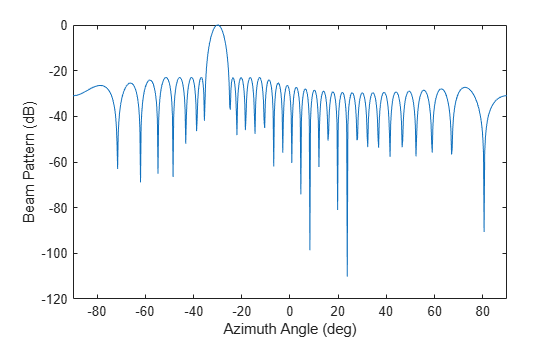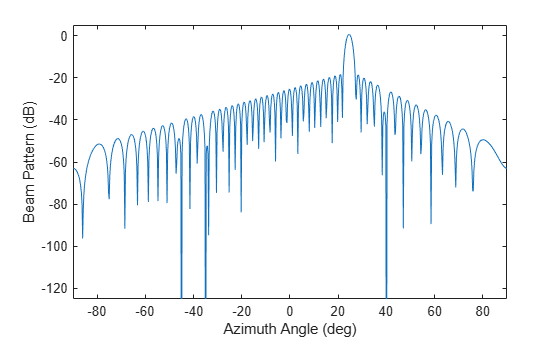# minvarweights

Weights for minimum-variance array pattern synthesis

## Syntax

``wts = minvarweights(pos,ang)``
``wts = minvarweights(pos,angcov)``
``wts = minvarweights(___,MaskAngle=angm)``
``wts = minvarweights(___,MaskSidelobeLevel=sllm)``
``wts = minvarweights(___,NullAngle=angn)``

## Description

example

````wts = minvarweights(pos,ang)` computes the minimum-variance weights `wts` for synthesizing the pattern of a sensor array in the directions specified by `ang`. Array element positions are specified in `pos`. The function optimizes the beamforming weights using a second-order cone programming solver.```
````wts = minvarweights(pos,angcov)` also specifies the spatial covariance matrix `cov` of the array elements.```

example

````wts = minvarweights(___,MaskAngle=angm)` also specifies angles `angm` at which mask sidelobe levels are defined in the `sllm` argument.```

example

````wts = minvarweights(___,MaskSidelobeLevel=sllm)` also specifies maximum allowable sidelobe levels `sllm` at the angles defined in `angm`.```

example

````wts = minvarweights(___,NullAngle=angn)` also specifies null directions `angn` for the array.```

## Examples

collapse all

Compute optimized beamforming weights of a 31-element half-wavelength spacing ULA in the direction of ${-30}^{\circ }$ degree in azimuth. Design the array to keep sidelobe levels less than -23 dB.

Create the optimized weights.

```N = 31; pos = (0:N-1)*0.5; sll = -23; wts = minvarweights(pos,-30,MaskSidelobeLevel=sll);```

Apply the optimized weights and display the array pattern from $-{90}^{\circ }$ to ${+90}^{\circ }$ azimuth.

```az = -90:.25:90; pat_opt = arrayfactor(pos,az,wts); plot(az,mag2db(abs(pat_opt))) xlabel('Azimuth Angle (deg)') ylabel('Beam Pattern (dB)') xlim([-90,90])```Design an array to have a tapered beampattern, The array is a 51-element half-wavelength spacing ULA steered in the direction of ${25}^{\circ }$ in azimuth. The pattern synthesis goal is to achieve sidelobe levels smaller than a tapered mask decreasing linearly from -18 dB to -55 dB at $±{90}^{\circ }$. Place nulls at ${-35}^{\circ }$, $-{45}^{\circ }$, and ${40}^{\circ }$ azimuth angle.

```N = 51; pos = (0:N-1)*0.5; ANGmainBeam = 25; angn = [-35 -45 40]; angm = [-90:.2:22 27:0.2:90]; sllm = [linspace(-55,-18,length(-90:.2:22)) ... linspace(-18,-55,length(27:.2:90))]; wts = minvarweights(pos,ANGmainBeam,'MaskAngle',angm, ... 'MaskSidelobeLevel',sllm,'NullAngle',angn);```

Apply optimized weights and display the array pattern from ${-90}^{\circ }$ to ${+90}^{\circ }$ in azimuth.

```az = -90:.25:90; pat_opt = arrayfactor(pos,az,wts); plot(az,mag2db(abs(pat_opt))) axis([-90 90 -125 5]) xlabel('Azimuth Angle (deg)') ylabel('Beam Pattern (dB)')```Verify that nulls are placed at ${-35}^{\circ }$, $-{45}^{\circ }$, and ${40}^{\circ }$ azimuth angle.

## Input Arguments

collapse all

Positions of the elements of a sensor array, specified as a 1-by-N vector, a 2-by-N matrix, or a 3-by-N matrix. In this vector or matrix, N represents the number of elements of the array. Each column of `pos` represents the coordinates of an element. If `pos` is a 1-by-N vector, then it represents the y-coordinate of the sensor elements of a line array. The x and z-coordinates are assumed to be zero. When `pos` is a 2-by-N matrix, it represents the (y,z)-coordinates of the sensor elements of a planar array. This array is assumed to lie in the yz-plane. The x-coordinates are assumed to be zero. When `pos` is a 3-by-N matrix, then the array can have an arbitrary shape. Sensor positions are in terms of signal wavelength.

Example: `[0,0,0; 0.1,0.4,0.3; 1,1,1]`

Data Types: `double`

Beamforming directions, specified as a 1-by-M vector or a 2-by-M matrix. In this vector or matrix, M represents the number of incoming signals. If `ang` is a 2-by-M matrix, each column specifies the direction in azimuth and elevation of the beamforming direction as `[az;el]`. Angular units are specified in degrees. The azimuth angle must lie between –180° and 180° and the elevation angle must lie between –90° and 90°. The azimuth angle is the angle between the x-axis and the projection of the beamforming direction vector onto the xy plane. The angle is positive when measured from the x-axis toward the y-axis. The elevation angle is the angle between the beamforming direction vector and xy-plane. It is positive when measured towards the positive z axis. If `ang` is a 1-by-M vector, then it represents a set of azimuth angles with the elevation angles assumed to be zero.

Example: `[45;10]`

Data Types: `double`

Sensor spatial covariance matrix, specified as an N-by-N complex-valued matrix. N is the number of array sensor elements.

Example: `[5,0.1;0.1,2]`

Data Types: `double`
Complex Number Support: Yes

Angles at which mask sidelobe levels are defined, specified as a real-valued 1-by-K vector or a real-valued 2-by-K matrix where K is the number of mask sidelobe levels. If `angm` is a 1-by-K vector, then it contains the azimuth angles of the mask directions. If `angm` is a 2-by-K matrix, each column specifies the direction in the form `[az;el]`. Angle units are in degrees.

Data Types: `double`

Maximum allowable mask sidelobe levels, specified as a non-positive scalar or non-positive real-valued 1-by-K vector. K is the number of mask sidelobe levels. Sidelobe levels are always less then or equal to zero.

• If `sllm` is a scalar, then it contains a uniform mask for all sidelobe levels and `angm` must be empty.

• If `sllm` is a 1-by-K vector, then `sllm` and `angm` must have the same number of columns; and `sllm` contains the mask sidelobe levels for corresponding mask angles, `angm`.

An empty `sllm` vector means that there are no constraints on the sidelobe levels. Units are in dB.

Data Types: `double`

Null direction angles, specified as either a 1-by-P vector or a 2-by-P matrix where P is the number of null directions. If `angn` is a 1-by-P vector, then it contains only the azimuth angles of directions. If `angn` is a 2-by-P matrix, each column specifies the null direction in the form `[az; el]`. Angle units are in degrees.

Data Types: `double`

## Output Arguments

collapse all

Beamformer weights, returned as a complex-valued N-by-1 vector. N represents the number of sensor elements of the array.

 Lebret, H., and S. Boyd. “Antenna Array Pattern Synthesis via Convex Optimization.” IEEE Transactions on Signal Processing, vol. 45, no. 3, Mar. 1997, pp. 526–32. DOI.org (Crossref), https://doi.org/10.1109/78.558465.

 Golbon-Haghighi, Mohammad-Hossein, et al. “Design of a Cylindrical Crossed Dipole Phased Array Antenna for Weather Surveillance Radars.” IEEE Open Journal of Antennas and Propagation, vol. 2, 2021, pp. 402–11. DOI.org (Crossref), https://doi.org/10.1109/OJAP.2021.3059471.